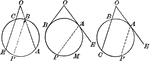### Circles With Angles Formed by Secants and Tangents

Illustration showing angles formed by two secants, two tangents, or a tangent and a secant, drawn to…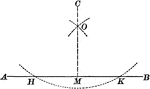### Construction of Perpendicular Upon a Given Line From an External Point

Illustration to let fall a perpendicular upon a given line from a given external point.### Parallel Tangents to an Ellipse

Illustration showing that tangents drawn at the ends of any diameter are parallel to each other.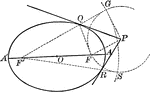### Tangent From External Point to an Ellipse

Illustration of how to draw a tangent to an ellipse from an external point.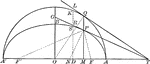### Tangents to an Ellipse

Illustration showing the tangents drawn at two corresponding points of an ellipse and its auxiliary…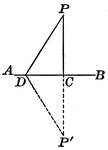### Perpendicular Line Drawn To a Given Line From an External Point

Illustration showing that the perpendicular is the shortest line that can be drawn to a straight line…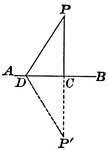### Perpendicular Line Drawn to a Given Line from an External Point

Illustration showing only one perpendicular can be drawn to a given line from a given external point.